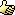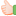CodeGuru Home VC++ / MFC / C++ .NET / C# Visual Basic VB Forums Developer.com

# Thread: Simplest way to find power of number...

1. ryuMemberJoin Date
Oct 2004
Posts
481

## Simplest way to find power of number...

Hi GURUs,

I want to be able to find out whether a specific number is 2^x or not.

For example:
64 --> yes, it is a 2^6
32 --> yes, it is a 2^5
24 --> no, because it is not part of 2 power of x.
8 --> yes, because it is 2^3
6 --> no, because it is not a 2 power of x.

My question is, given a specific a number, how to determine if it is a 2^x value or not?

I know the hard way which is do a loop until the value we want to find is greater than 2^x.

Is there a more simple way to do it?

CheersReply With Quote

2. ## Re: Simplest way to find power of number...

i would just keep dividing the number by 2, and see if i end with a 1. However, i bet you could check the bits of the number, since they should all be a "1" if it is a power of 2. not sure how to write that code exactly though sorry :PReply With Quote

3. ryuMemberJoin Date
Oct 2004
Posts
481

## Re: Simplest way to find power of number...

Hi Bovinedragon,

I know how to code to check the binary number. However, I can't workout the logic. Do you know the logic to do the binary check?

All the number power by two is always have 1 and only 1 in the binary number.
eg.
2 --> 1
4 --> 10
8 --> 100
16 --> 1000
etc...
But what is the "magic" number so I can do bitwise operation?

CheersReply With Quote

4. ## Re: Simplest way to find power of number...

Hello,

I am afraid that there is no ready made solutions for this problem. Simplest I can think of is masking the number with powers of 2, like 1, 2, 4, 8 etc till the highest power of 2 below the specified number and add all the resulting numbers. If the sum is 1, it is a power of 2. Otherwise it is a combination of powers of 2. What we do precisaely is to check the 1s in the bit pattern of that number.

Another method is the check for equality to all the powers of 2 below the specified number. If the number is not equal to any of the powers of 2, it is a combination of powers of 2. But I feel that this will be less efficient in terms of execution since checking equality in each running of the loop is not an optimized option.

Here is a sample code which returns true if the parameter is a power of 2. I am using the first method.

Code:
```bool ispowerof2(int number)
{
for (int power = 1, ones = 0; power <= number; power <<= 1)
ones += (power & number ? 1 : 0);
return ones == 1;
}```
Regards,
Pravin.Reply With Quote

5.Senior MemberJoin Date
May 2006
Location
Norway
Posts
1,709

## Re: Simplest way to find power of number...

I think this one is far better:
Code:
```bool IsPower2(int x)
{
return ( (x > 0) && ((x & (x - 1)) == 0) );
}```
LaitinenReply With Quote

6. ## Re: Simplest way to find power of number...

Great. I didn't know this one.Reply With Quote

7.MemberJoin Date
Oct 2005
Posts
199

## Re: Simplest way to find power of number...

I would have shifted given number right until rightmost bit is '1'. If result is one, number is power of 2.Reply With Quote

8. ## Re: Simplest way to find power of number...Originally Posted by laitinen
I think this one is far better:
Code:
```bool IsPower2(int x)
{
return ( (x > 0) && ((x & (x - 1)) == 0) );
}```
Laitinen
Wow! Really great. Only for powers of 2, that number and its predicissor will AND to give 0. By this method, any calculation in loop is avoided.

Hats off to laitinen.

Regards,
Pravin.Reply With Quote

9. ryuMemberJoin Date
Oct 2004
Posts
481

##Re: Simplest way to find power of number...

Hi Laitinen,

Thanks for sharing the algorithm! I could never though of that. Very simple solution.

Thanks again...

CheersReply With Quote

####Posting Permissions

• You may not post new threads
• You may not post replies
• You may not post attachments
• You may not edit your posts
•